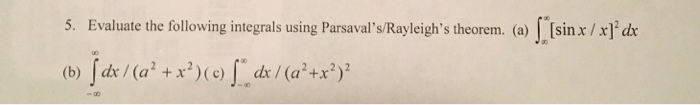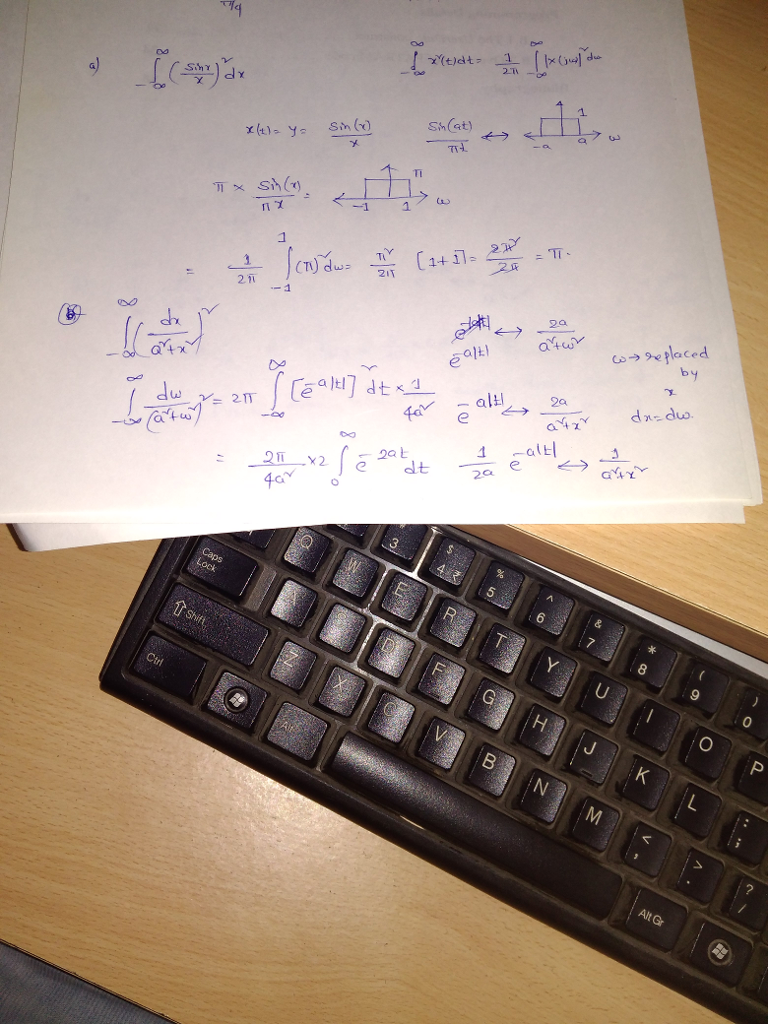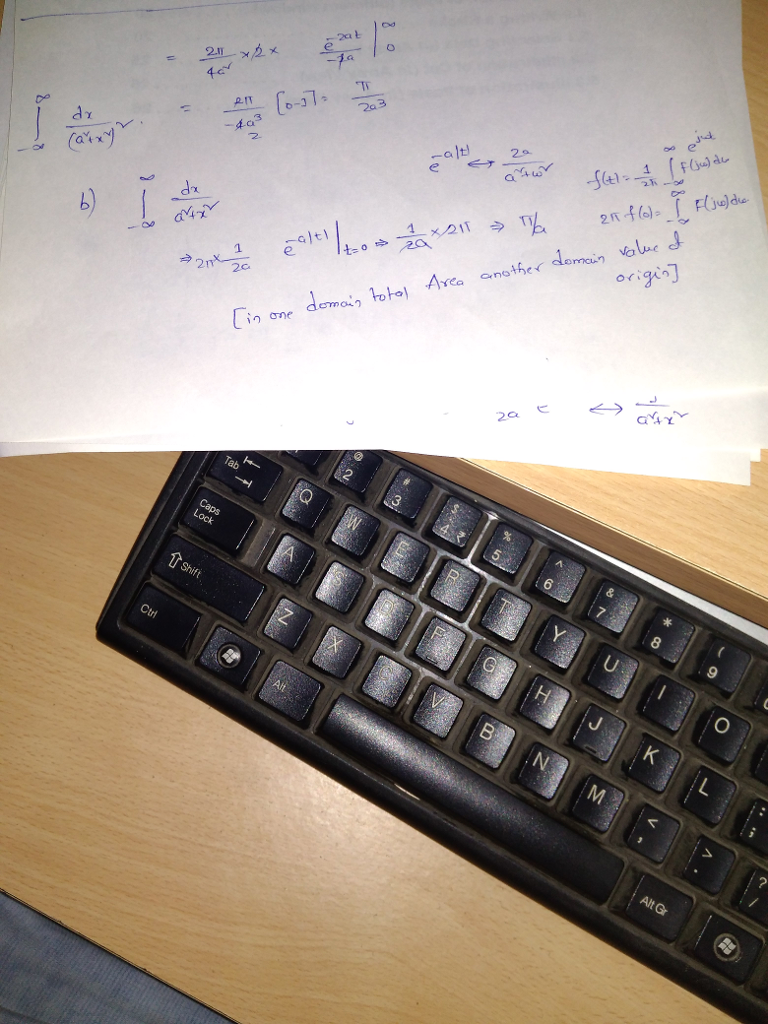# Homework Solution: Evaluate the following integrals using Parsaval's/Rayleigh's theorem. (a) interal_infinity^infinity [sinx/x]^2 dx (b) integral_ – i…Evaluate the following integrals using Parsaval's/Rayleigh's theorem. (a) interal_infinity^infinity [sinx/x]^2 dx (b) integral_ - infinity^infinity dx/(a^2 + x^2) (c) integral_ - infinity^infinity dx/(a^2 + x^2)^2